### Concept of Addition – Questions

Q.1) Count the objects in the Two boxes and select the right answer?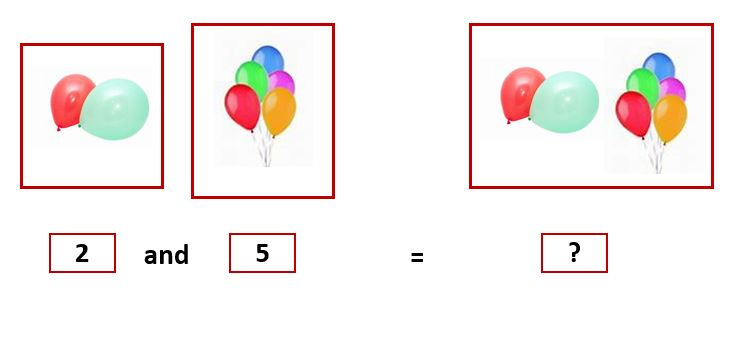[A] ​7

[B] ​4

Q.2) Count the objects in the Two boxes and select the right answer?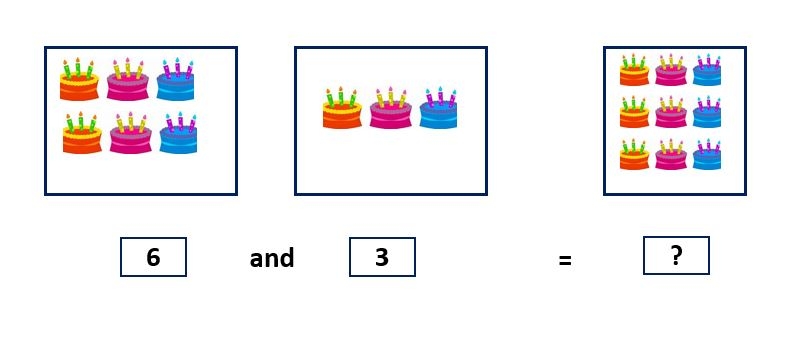[A] ​7

[B] ​9

Q.3) Count the objects in the Two boxes and select the right answer?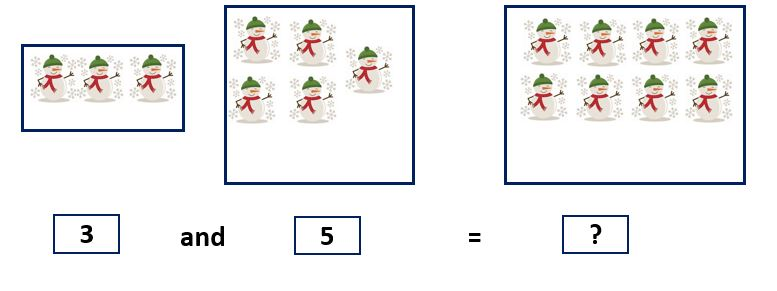[A] ​8

[B] ​5

Q.4) Count the objects in the Two boxes and select the right answer?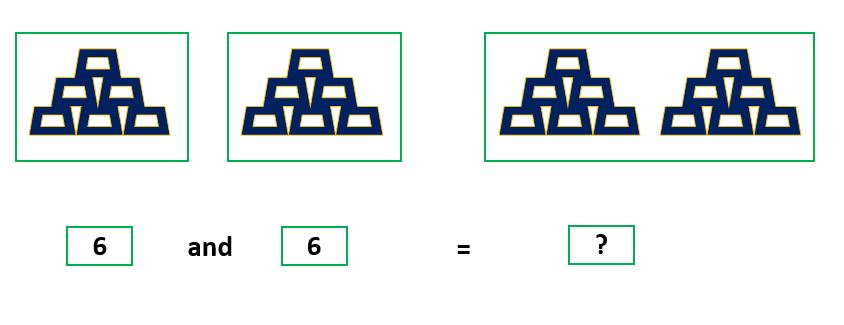[A] ​12

[B] ​11

Q.5) Count the objects in the Two boxes and select the right answer?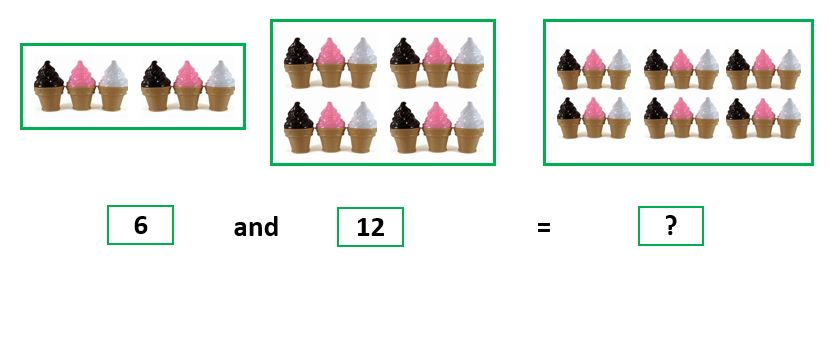[A] ​17

[B] ​18

Q.6) Count the objects in the Two boxes and select the right answer?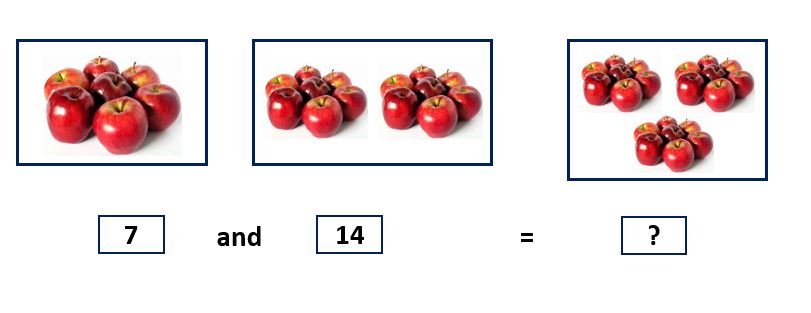[A] ​21

[B] ​27

Q.7) Count the objects in the Two boxes and select the right answer?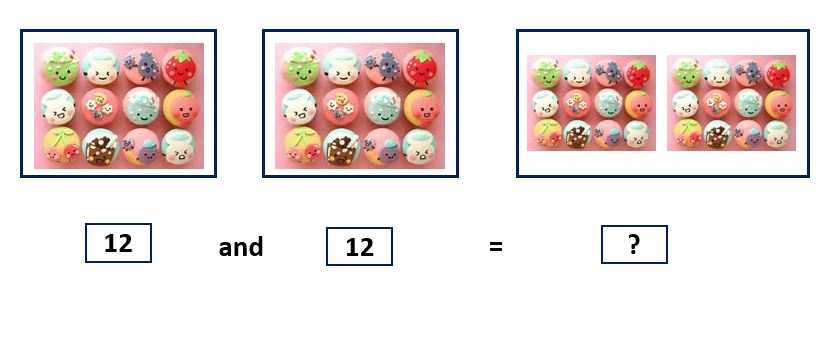[A] ​18

[B] ​24

Q.8) Count the objects in the Two boxes and select the right answer?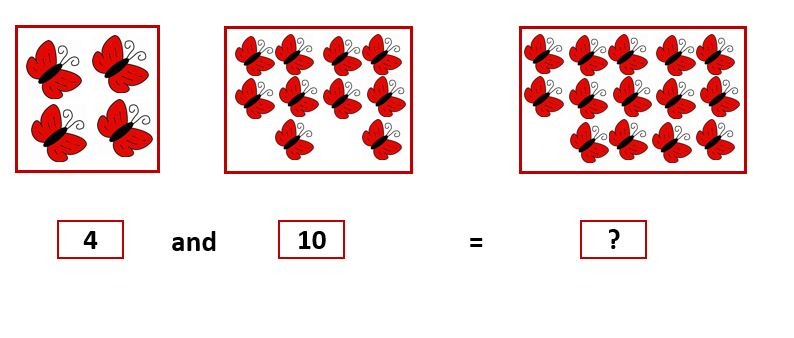[A] ​14

[B] ​12

Q.9) Count the objects in the Two boxes and select the right answer?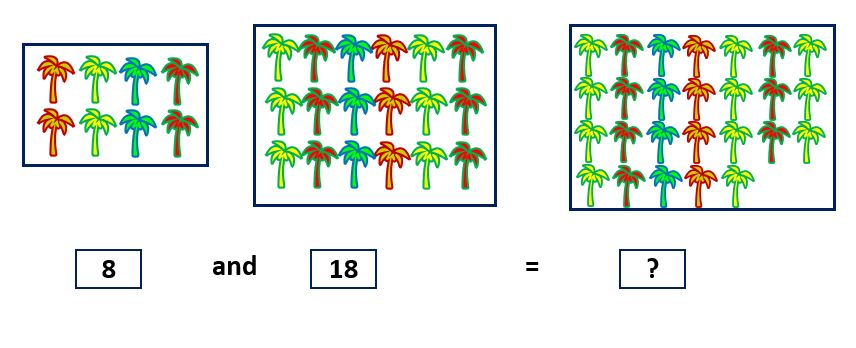[A] ​26

[B] ​28

Q.10) Count the objects in the Two boxes and select the right answer?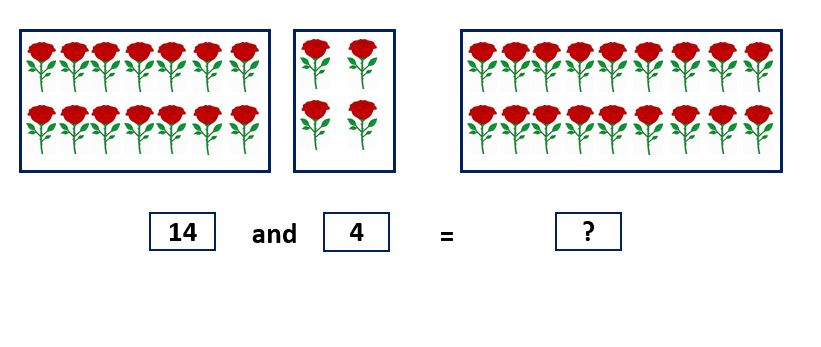[A] ​15

[B] ​18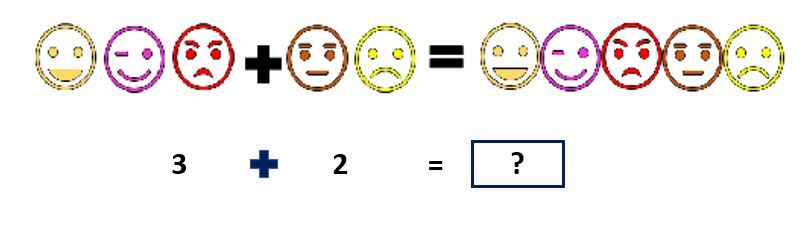​[A] 5​

[B] ​7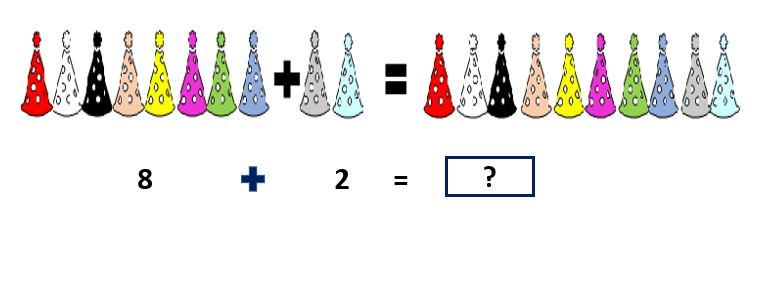[A] ​8

[B] ​10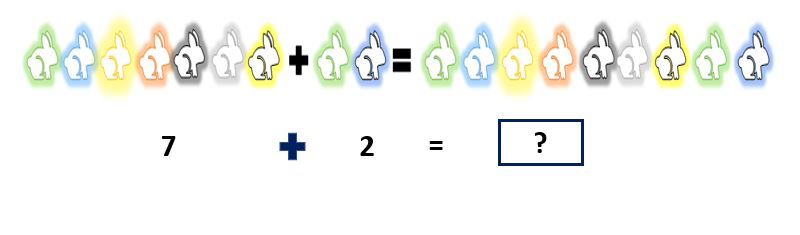[A] ​9

[B] ​12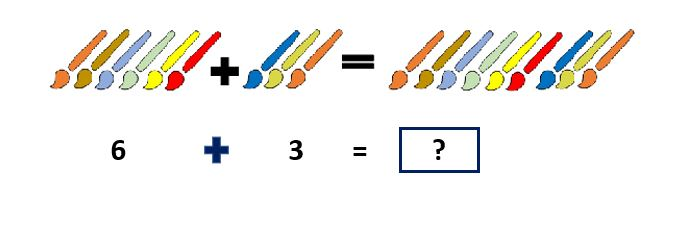[A] ​13

[B] ​9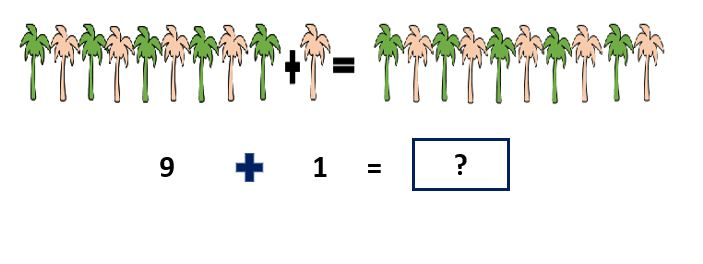[A] ​10

[B] ​13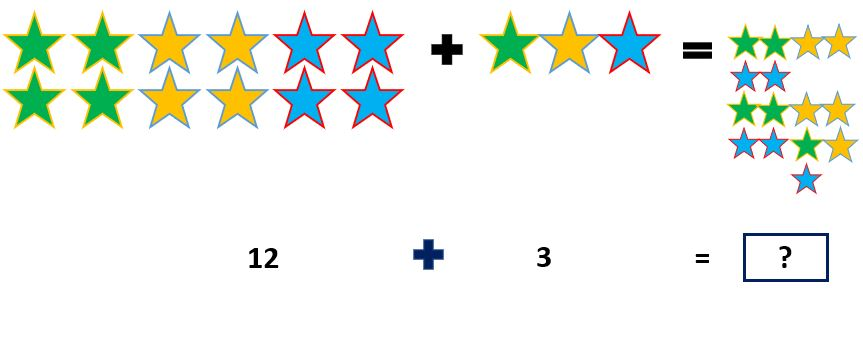[A] ​17

[B] ​15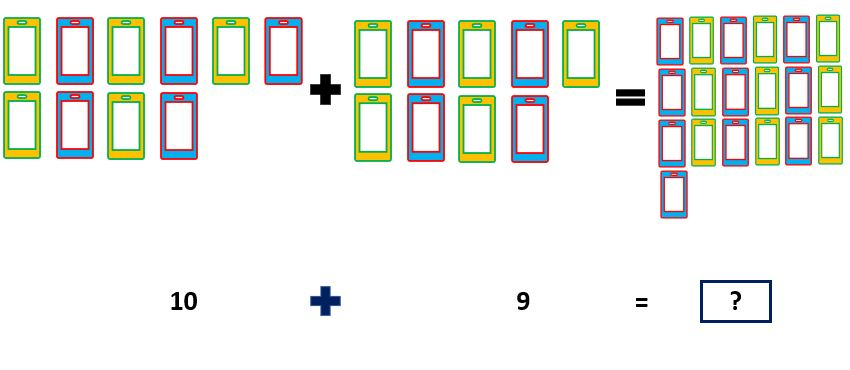​[A] ​19

[B] ​22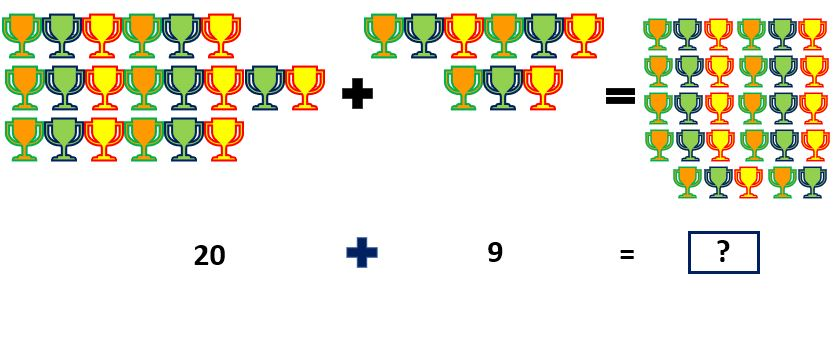[A] ​32

[B] ​29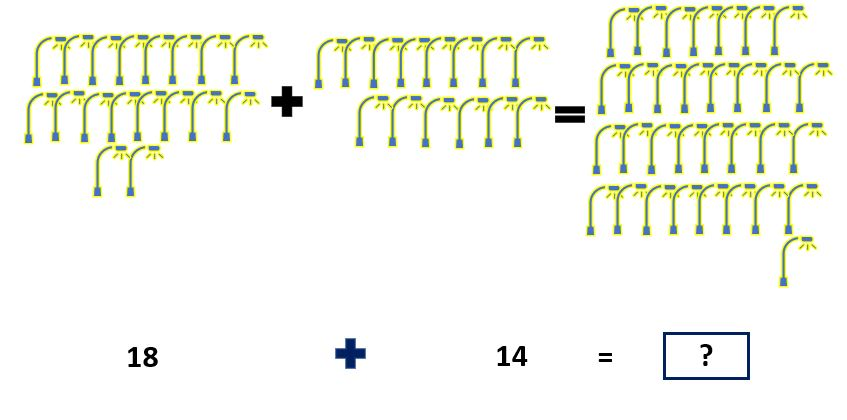​[A] ​32

[B] ​38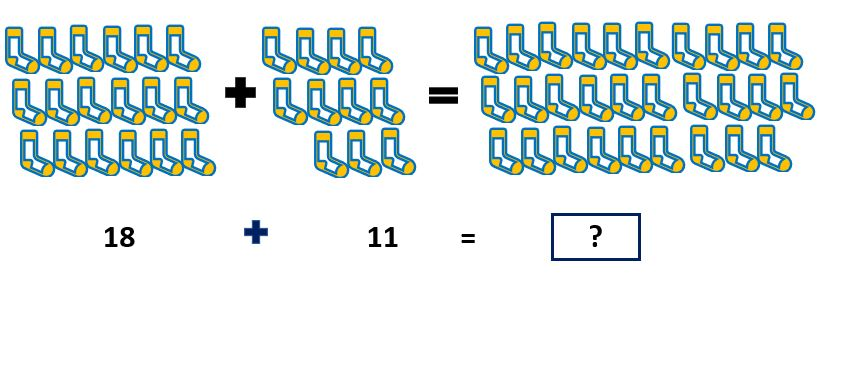[A] ​35

[B] ​29

### Missing Number 1 – Questions – Addition Worksheets for Grade 1

Q.1) ​Write the missing number?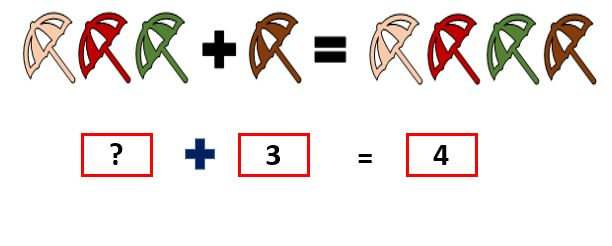[A] ​1

[B] ​2

Q.2) ​​Write the missing number?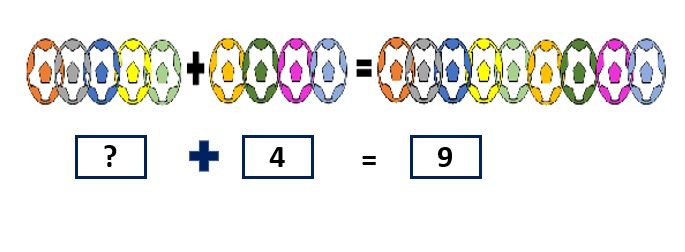​[A] ​4

[B] ​5

Q.3) ​​Write the missing number?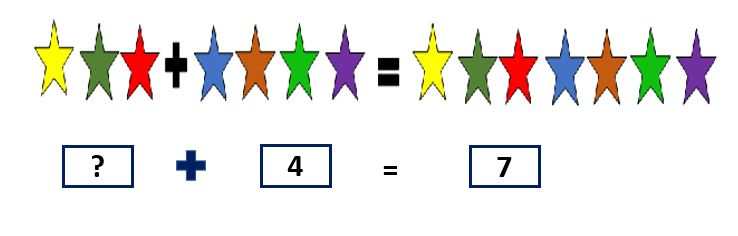​[A] ​3

[B] ​1

Q.4) ​​Write the missing number?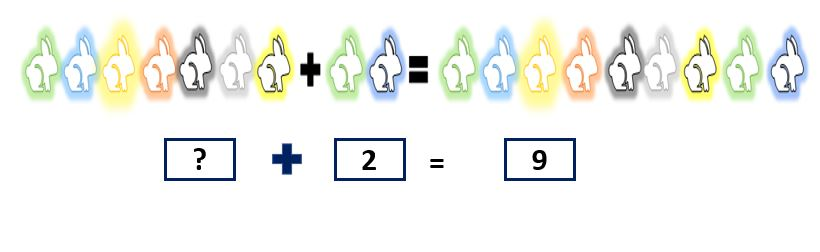​[A] ​7

[B] ​5

Q.5) ​​Write the missing number?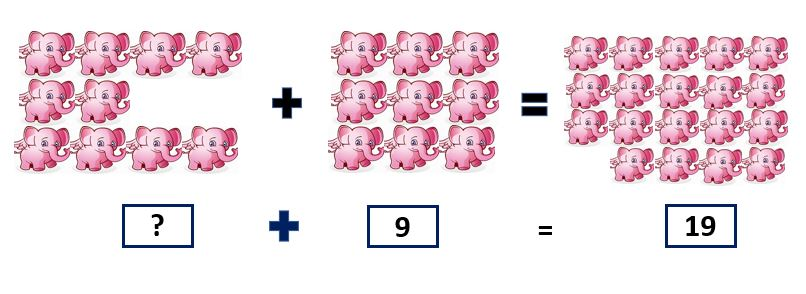​[A] ​10

[B] ​13

Q.6) ​​Write the missing number?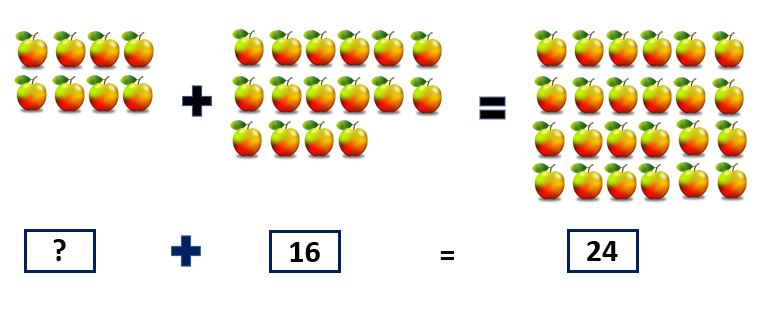​[A] 8​

[B] ​16

Q.7) ​​Write the missing number?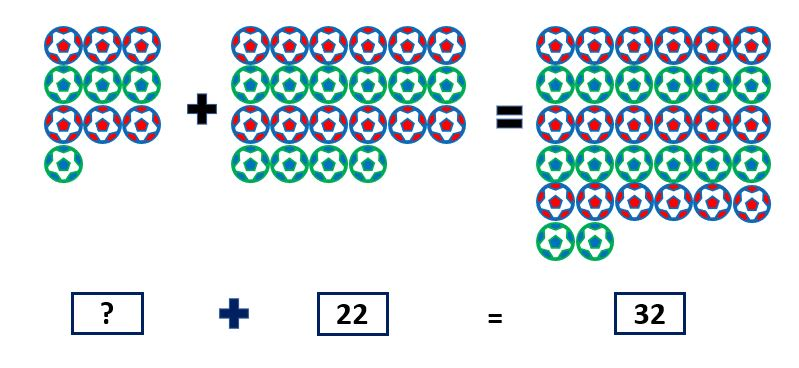​[A] ​10

[B] 12​

Q.8) ​​Write the missing number?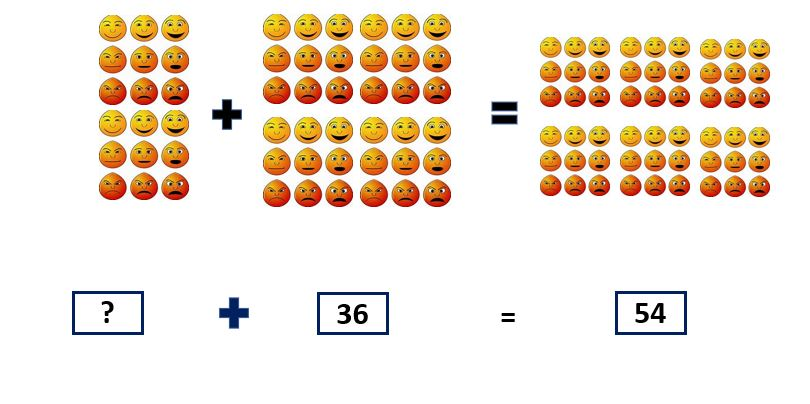[A] ​22

[B] ​18

Q.9) ​​Write the missing number?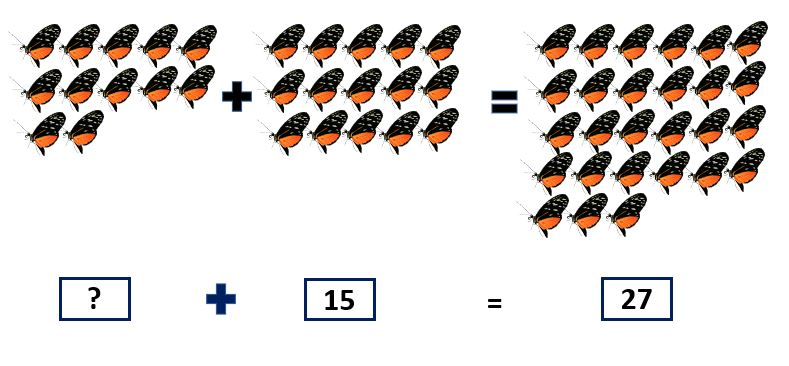​[A] ​12

[B] ​15

Q.10) ​​Write the missing number?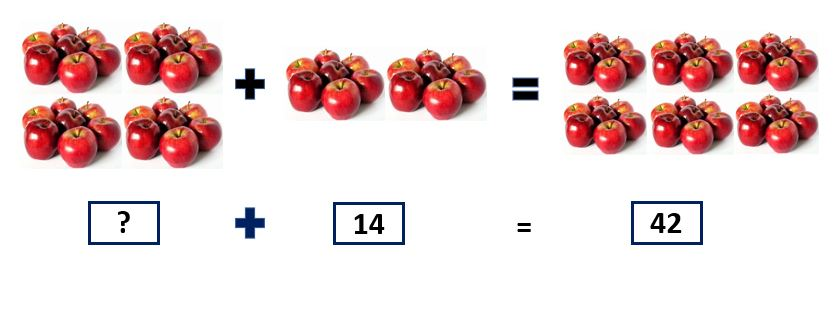[A] ​35

[B] ​28

Q.1) ​​Choose the correct addition statement for the image?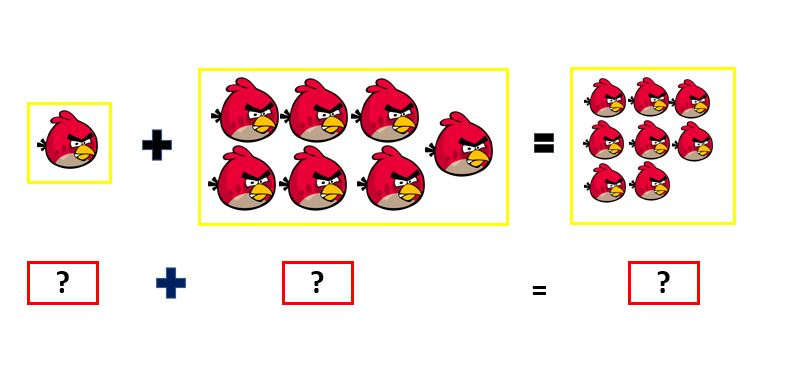​[A] ​1+7=8

[B] ​1+6=7

Q.2) ​Choose the correct addition statement for the image?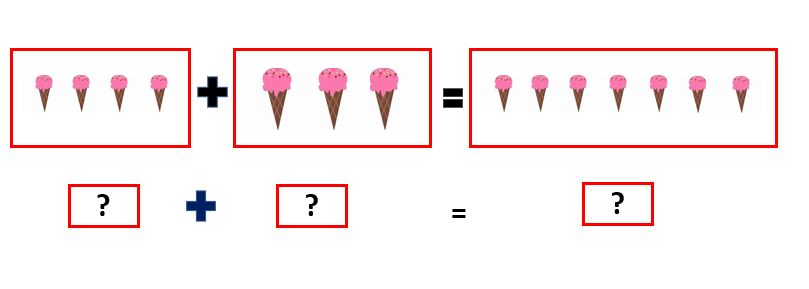​[A] ​3+2=5

[B] ​4+3=7

Q.3) ​Choose the correct addition statement for the image?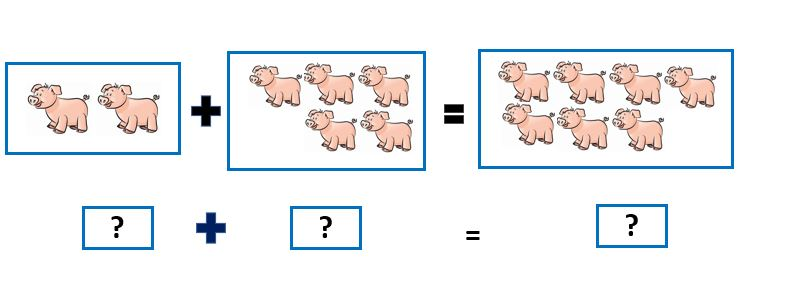​[A] ​2+5=7

[B] ​3+5=8

Q.4) ​Choose the correct addition statement for the image?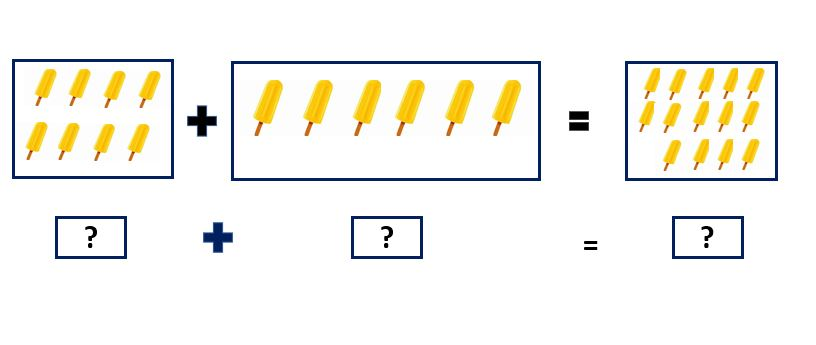[A] ​6+5=11

[B] ​8+6=14

Q.5) ​Choose the correct addition statement for the image?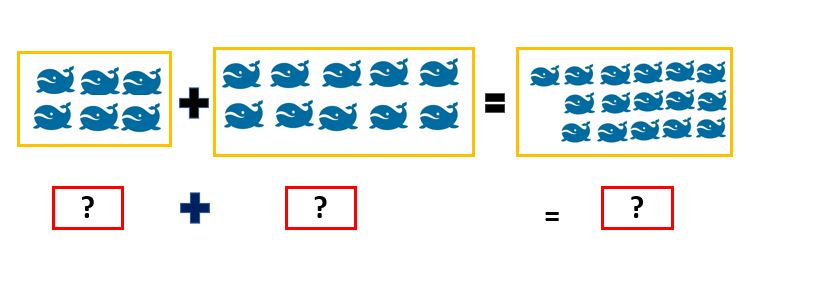[A] ​6+10=16

[B] ​5+9=14

Q.6) ​Choose the correct addition statement for the image?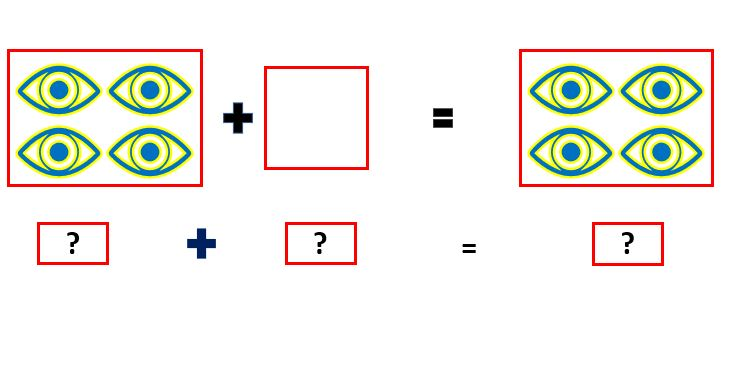[A] ​4+0=4

[B] ​3+0=3

Q.7) ​Choose the correct addition statement for the image?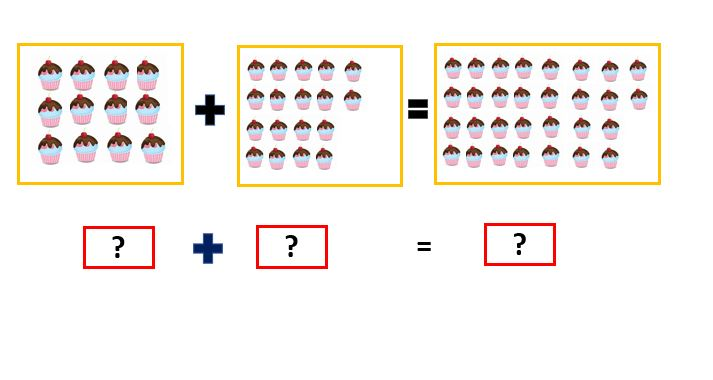[A] ​12+16=28

[B] ​12+18=30

Q.8) ​​Choose the correct addition statement for the image?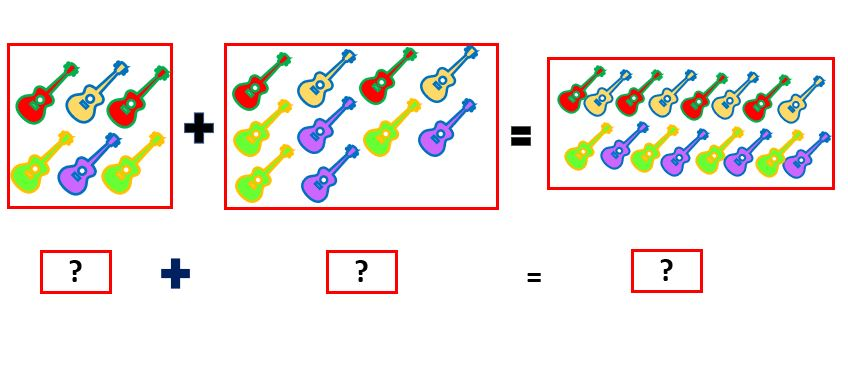[A] ​6+10=16

[B] ​10+3=13

Q.9) ​​Choose the correct addition statement for the image?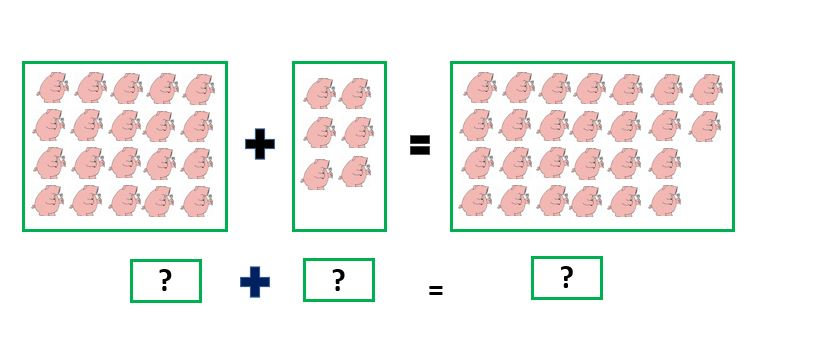​[A] ​18+4=22

[B] ​20+6=26

Q.10) ​​Choose the correct addition statement for the image?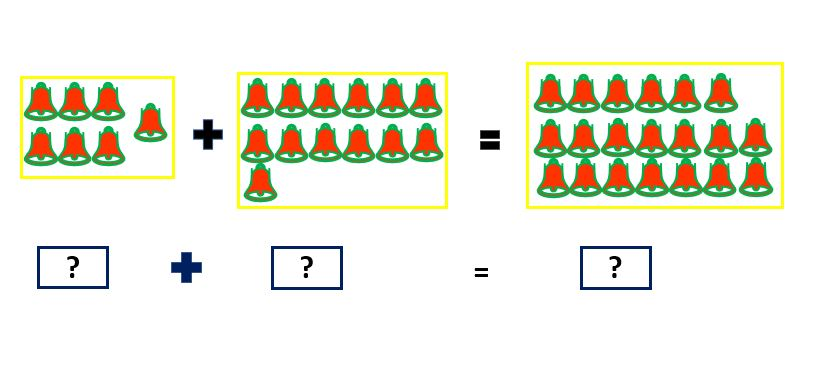[A] ​7+13=20

[B] ​5+7=12

Q.1)A Q.2)B Q.3)A Q.4)A Q.5)B Q.6)A Q.7)B Q.8)A Q.9)A Q.10)B